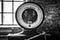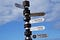# Weighted Linear RegressionPhoto by Jason on Unsplash

Linear regression is one of the simplest and well-known supervised machine learning models. In linear regression, the response variable (dependent variable) is modeled as a linear function of features (independent variables). Linear regression relies on several important assumptions which cannot be satisfied in some applications. In this article, we look into one of the main pitfalls of linear regression: heteroscedasticity.

# Linear Regression Model

We start with the linear regression mathematical model. Assume there are m observations and n features. The linear regression model is expressed as

# Fast Distance Calculation in PythonPhoto by Bruno on Unsplash

In many machine learning applications, we need to calculate the distance between two points in an Euclidean space. For example, in the k-nearest neighbors (k-NN) algorithm, we need to find the distance between each point in the test dataset to all points in the train dataset and select the train points with smallest distances. In the k-means clustering, the distance between each observation and all cluster centers should be calculated during assignment (association) stage. Therefore, a fast and efficient distance calculation algorithm is highly required.

# Distance in Euclidean Space

The distance between two points in an Euclidean space Rⁿ can be calculated using p-norm…## Reza Vaghefi

PhD in ECE | Data Scientist | Coding Enthusiast, https://www.linkedin.com/in/reza-vaghefi-b1829516/